# Minimum/Maximum Falling Path Sum (DP-12)

In this article, we will solve the most asked coding interview problem: Minimum/Maximum falling path sum.

Problem Link: Variable Starting and Ending Point

Problem Description:

We are given an ‘N*M’ matrix. We need to find the maximum path sum from any cell of the first row to any cell of the last row.

At every cell we can move in three directions: to the bottom cell (↓), to the bottom-right cell(↘), or to the bottom-left cell(↙).

```Example: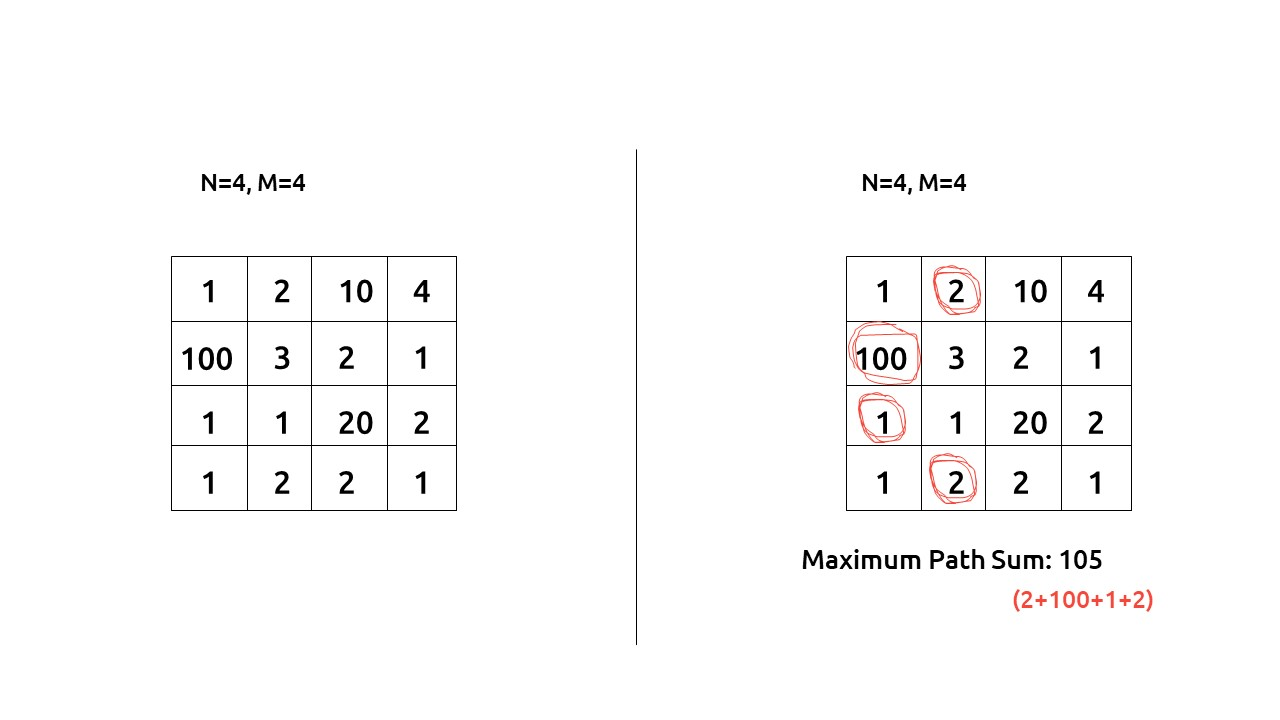```

Disclaimer: Don’t jump directly to the solution, try it out yourself first.

### Solution :

This question is a slight modification of the question discussed in Minimum Path Sum in a Triangular Grid. In the previous problem, we were given a fixed starting and a variable ending point, whereas here the problem states that the starting point can be any cell from the first row and the ending point can be any cell in the last row.

Why a Greedy Solution doesn’t work?

As we have to return the minimum path sum, the first approach that comes to our mind is to take a greedy approach and always form a path by locally choosing the cheaper option. But there is no ‘uniformity’ in the values of the string, therefore it can happen that whenever we are making a local choice that gives us a better path, we actually take a path which in the later stages is giving us the lesser path sum.

As a greedy solution doesn’t work, our next choice will be to try out all the possible paths. To generate all possible paths we will use recursion.

Steps to form the recursive solution:

We will first form the recursive solution by the three points mentioned in Dynamic Programming Introduction

Step 1: Express the problem in terms of indexes.

We are given an ‘N*M’ matrix. We can define the function with two parameters i and j, where i and j represent the row and column of the matrix.

Now our ultimate aim is to reach the last row. We can define f(i,j) such that it gives us the maximum path sum from any cell in the first row to the cell[i][j].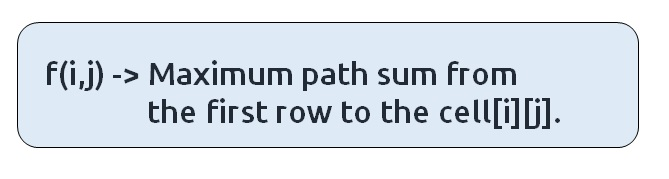If we see the figure given below: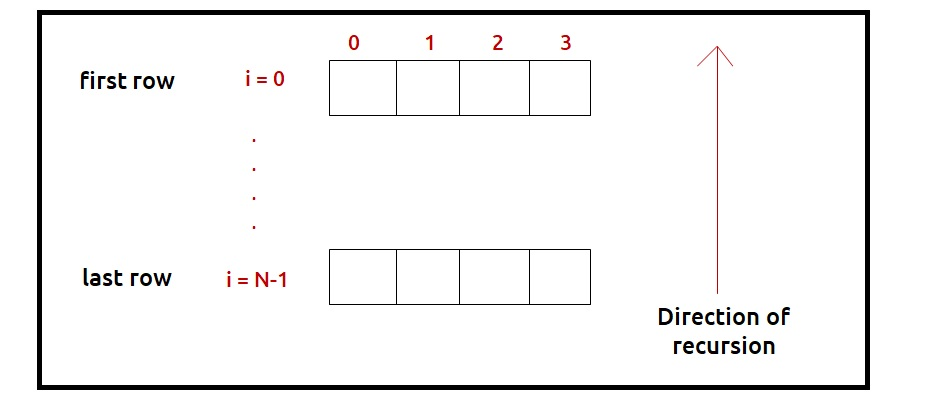We have a top row and a bottom row, we will be writing a recursion in the direction of the last row to the first row. For the last row, i=N-1 therefore we need to find four different answers:

f(N-1,0), f(N-1,1), f(N-1,2), f(N-1,3)

These recursive calls will give the maximum path sum from a cell in the first row to the respective four cells for which the recursion calls are made. We need to return the maximum value among these as the final answer.

Base Case:

There will be the following base cases:

• When i == 0, it means we are at the first row, so the min path from that cell to the first will be the value of that cell itself, hence we return mat[j].

At every cell we have three options (we are writing recursion from the last row to the first row): to the top cell (↑), to the top-right cell(↗), or to the top-left cell(↖).

As we are moving to the top cell (↑), at max we will reach the first row, from where we return, so we will never go out of the bounding index.

To move to the top-left cell(↖) or to the top-right cell(↗), it can happen that we may go out of bound as shown in the figure(below). So we need to handle it, we can return -1e9, whenever we go out of bound, in this way this path will not be selected by the calling function as we have to return the maximum path.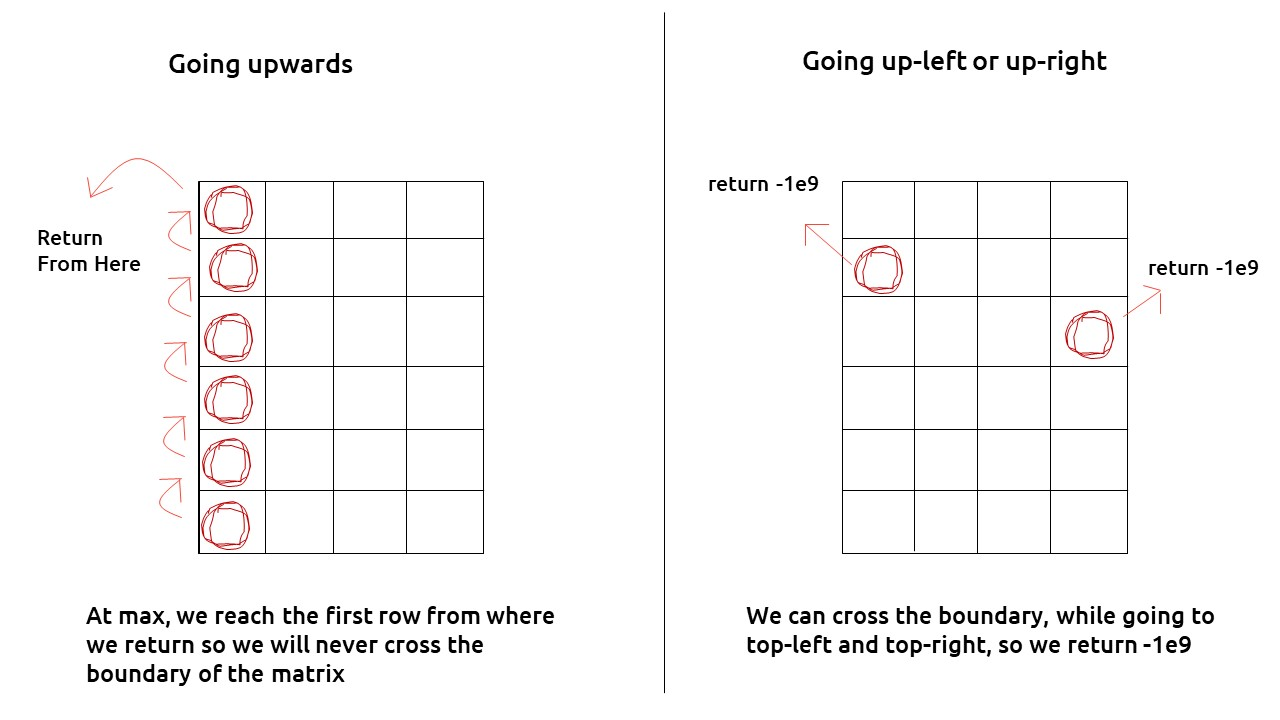• If j<0 or j>=M , then we return -1e9

The pseudocode till this step will be: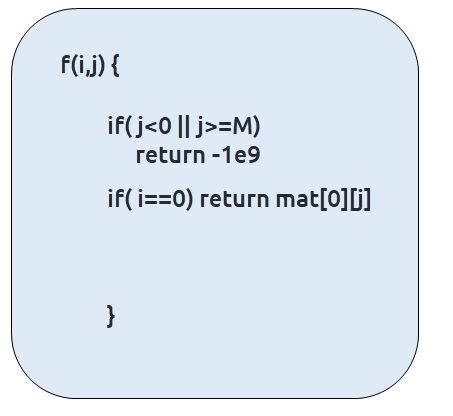Step 2: Try out all possible choices at a given index.

At every cell we have three options (we are writing recursion from the last row to the first row): to the top cell (↑), to the top-right cell(↗), or to the top-left cell(↖).

To go to the top, we will decrease i by 1, and to move towards top-left, we will decrease both i and j by 1 whereas to move to top-right, we will decrease i by 1 and increase j by 1.

Now when we get our answer for the recursive call (f(i-1,j), f(i-1,j-1) or f(i-1,j+1)), we need to also add the current cell value to it as we have to include it too for the current path sum.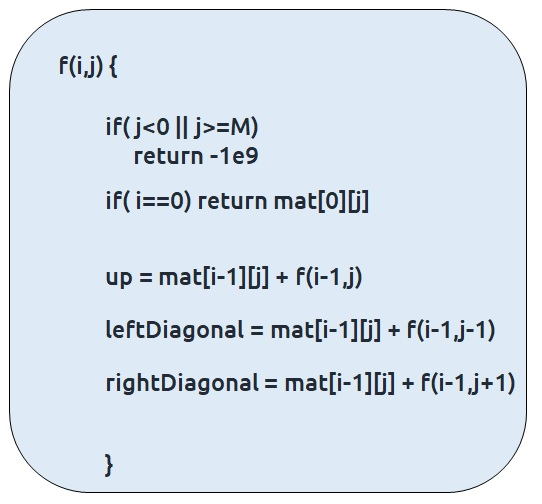Step 3:  Take the maximum of all choices

As we have to find the maximum path sum of all the possible unique paths, we will return the maximum of all the choices(up, leftDiagonal, right diagonal)

The final pseudocode after steps 1, 2, and 3: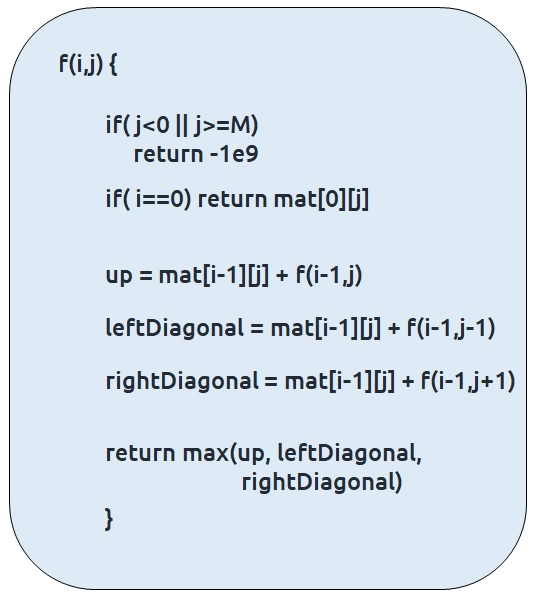Steps to memoize a recursive solution:

If we draw the recursion tree, we will see that there are overlapping subproblems. In order to convert a recursive solution the following steps will be taken:

1. Create a dp array of size [N][M]
2. Whenever we want to find the answer of a particular row and column (say f(i,j)), we first check whether the answer is already calculated using the dp array(i.e dp[i][j]!= -1 ). If yes, simply return the value from the dp array.
3. If not, then we are finding the answer for the given values for the first time, we will use the recursive relation as usual but before returning from the function, we will set dp[i][j] to the solution we get.

Code:

## C++ Code

``````#include <bits/stdc++.h>

using namespace std;

int getMaxUtil(int i, int j, int m, vector<vector<int>> &matrix,
vector<vector<int> > &dp){

// Base Conditions
if(j<0 || j>=m)
return -1e9;
if(i==0)
return matrix[j];

if(dp[i][j]!=-1) return dp[i][j];

int up = matrix[i][j] + getMaxUtil(i-1,j,m,matrix,dp);
int leftDiagonal = matrix[i][j] + getMaxUtil(i-1,j-1,m,matrix,dp);
int rightDiagonal = matrix[i][j] + getMaxUtil(i-1,j+1,m,matrix,dp);

return dp[i][j]= max(up,max(leftDiagonal,rightDiagonal));

}

int getMaxPathSum(vector<vector<int> > &matrix){

int n = matrix.size();
int m = matrix.size();

vector<vector<int>> dp(n,vector<int>(m,-1));

int maxi = INT_MIN;

for(int j=0; j<m;j++){
int ans = getMaxUtil(n-1,j,m,matrix,dp);
maxi = max(maxi,ans);
}

return maxi;
}

int main() {

vector<vector<int> > matrix{{1,2,10,4},
{100,3,2,1},
{1,1,20,2},
{1,2,2,1}};

cout<<getMaxPathSum(matrix);
}
``````

Output:

105

Time Complexity: O(N*N)

Reason: At max, there will be M*N calls of recursion to solve a new problem,

Space Complexity: O(N) + O(N*M)

Reason: We are using a recursion stack space: O(N), where N is the path length and an external DP Array of size ‘N*M’.

## Java Code

``````import java.util.*;

class TUF{

static int getMaxUtil(int i, int j, int m, int[][] matrix,int[][] dp){

// Base Conditions
if(j<0 || j>=m)
return (int)Math.pow(-10,9);
if(i==0)
return matrix[j];

if(dp[i][j]!=-1) return dp[i][j];

int up = matrix[i][j] + getMaxUtil(i-1,j,m,matrix,dp);
int leftDiagonal = matrix[i][j] + getMaxUtil(i-1,j-1,m,matrix,dp);
int rightDiagonal = matrix[i][j] + getMaxUtil(i-1,j+1,m,matrix,dp);

return dp[i][j]= Math.max(up,Math.max(leftDiagonal,rightDiagonal));

}

static int getMaxPathSum(int[][] matrix){

int n = matrix.length;
int m = matrix.length;

int dp[][]= new int[n][m];
for(int row[]: dp)
Arrays.fill(row,-1);

int maxi = Integer.MIN_VALUE;

for(int j=0; j<m;j++){
int ans = getMaxUtil(n-1,j,m,matrix,dp);
maxi = Math.max(maxi,ans);
}

return maxi;
}

public static void main(String args[]) {

int matrix[][] = {{1,2,10,4},
{100,3,2,1},
{1,1,20,2},
{1,2,2,1}};

System.out.println(getMaxPathSum(matrix));
}
}``````

Output:

105

Time Complexity: O(N*N)

Reason: At max, there will be M*N calls of recursion to solve a new problem,

Space Complexity: O(N) + O(N*M)

Reason: We are using a recursion stack space: O(N), where N is the path length and an external DP Array of size ‘N*M’.

Steps to convert Recursive Solution to Tabulation one.

The steps to convert to the tabular solution are given below:

• Declare a dp[] array of size [N][M].
• First initialize the base condition values, i.e the first row of the dp array to the first row of the input matrix.
• We want to move from the first row to the last row.Whenever we compute values for a cell, we want to have all the values required to calculate it.
• If we see the memoized code, values required for dp[i][j] are: dp[i-1][j], dp[i-1][j-1] and dp[i-1][j+1]. So we only need the values from the ‘i-1’ row.
• We have already filled the first row (i=0), if we start from row ‘1’ and move downwards we will find the values correctly.
• We can use two nested loops to have this traversal.
• At last we need to return the maximum among the last row of dp array as our answer.

Code:

## C++ Code

``````#include <bits/stdc++.h>

using namespace std;

int getMaxPathSum(vector<vector<int> > &matrix){

int n = matrix.size();
int m = matrix.size();

vector<vector<int>> dp(n,vector<int>(m,0));

// Initializing first row - base condition
for(int j=0; j<m; j++){
dp[j] = matrix[j];
}

for(int i=1; i<n; i++){
for(int j=0;j<m;j++){

int up = matrix[i][j] + dp[i-1][j];

int leftDiagonal= matrix[i][j];
if(j-1>=0) leftDiagonal += dp[i-1][j-1];
else leftDiagonal += -1e9;

int rightDiagonal = matrix[i][j];
if(j+1<m) rightDiagonal += dp[i-1][j+1];
else rightDiagonal += -1e9;

dp[i][j] = max(up, max(leftDiagonal,rightDiagonal));

}
}

int maxi = INT_MIN;

for(int j=0; j<m;j++){
maxi = max(maxi,dp[n-1][j]);
}

return maxi;
}

int main() {

vector<vector<int> > matrix{{1,2,10,4},
{100,3,2,1},
{1,1,20,2},
{1,2,2,1}};

cout<<getMaxPathSum(matrix);
}
``````

Output:

105

Time Complexity: O(N*M)

Reason: There are two nested loops

Space Complexity: O(N*M)

Reason: We are using an external array of size ‘N*M’. The stack space will be eliminated.

## Java Code

``````import java.util.*;

class TUF{

static int getMaxPathSum(int[][] matrix){

int n = matrix.length;
int m = matrix.length;

int dp[][] = new int[n][m];

// Initializing first row - base condition
for(int j=0; j<m; j++){
dp[j] = matrix[j];
}

for(int i=1; i<n; i++){
for(int j=0;j<m;j++){

int up = matrix[i][j] + dp[i-1][j];

int leftDiagonal= matrix[i][j];
if(j-1>=0) leftDiagonal += dp[i-1][j-1];
else leftDiagonal += (int)Math.pow(-10,9);

int rightDiagonal = matrix[i][j];
if(j+1<m) rightDiagonal += dp[i-1][j+1];
else rightDiagonal += (int)Math.pow(-10,9);

dp[i][j] = Math.max(up, Math.max(leftDiagonal,rightDiagonal));

}
}

int maxi = Integer.MIN_VALUE;

for(int j=0; j<m;j++){
maxi = Math.max(maxi,dp[n-1][j]);
}

return maxi;
}

public static void main(String args[]) {

int matrix[][] = {{1,2,10,4},
{100,3,2,1},
{1,1,20,2},
{1,2,2,1}};

System.out.println(getMaxPathSum(matrix));
}
}``````

Output:

105

Time Complexity: O(N*M)

Reason: There are two nested loops

Space Complexity: O(N*M)

Reason: We are using an external array of size ‘N*M’. The stack space will be eliminated.

Part 3: Space Optimization

If we closely look the relation,

dp[i][j] = matrix[i][j] + max(dp[i-1][j],dp[i-1][j-1], dp[i-1][j+1]))

We see that we only need the previous row, in order to calculate dp[i][j]. Therefore we can space optimize it.

Initially we can take a dummy row ( say prev). We initialize this row to the input matrix first row( as done in tabulation).

Now the current row(say cur) only needs the prev row’s value inorder to calculate dp[i][j].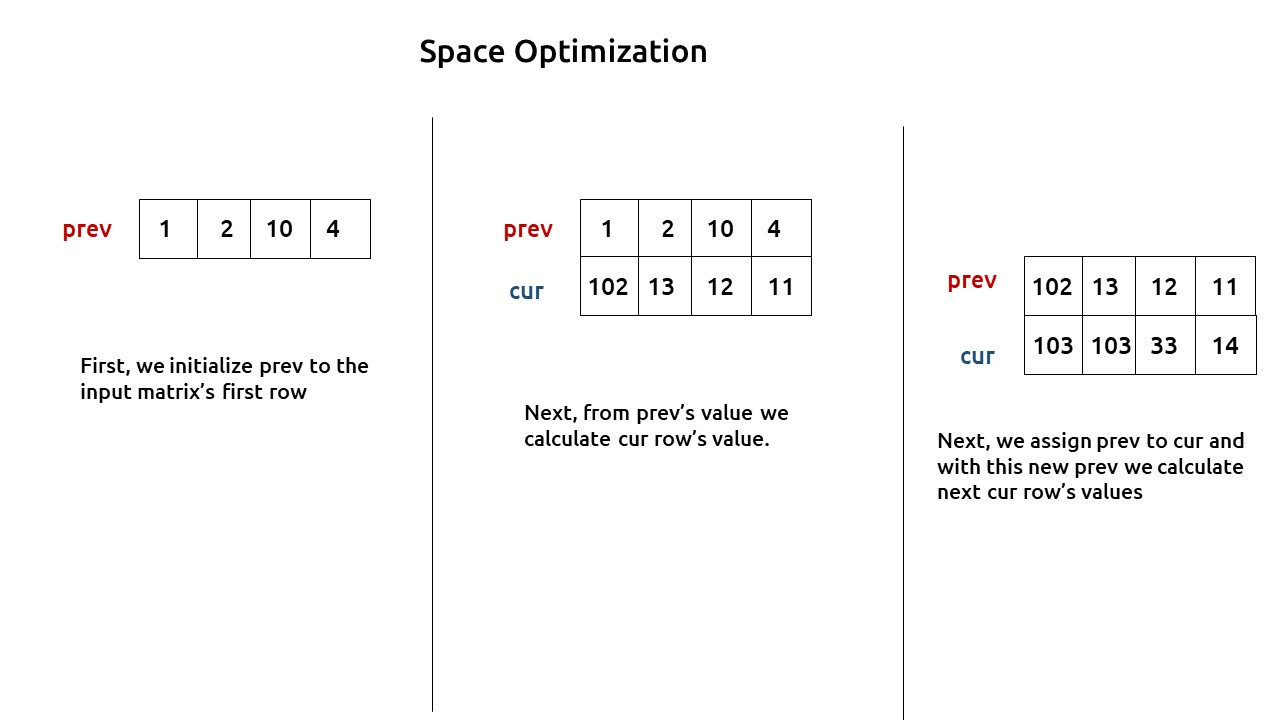At last, we will return the maximum value among the values of the prev row as our answer.

Code:

## C++ Code

``````#include <bits/stdc++.h>

using namespace std;

int getMaxPathSum(vector<vector<int> > &matrix){

int n = matrix.size();
int m = matrix.size();

vector<int> prev(m,0), cur(m,0);

// Initializing first row - base condition
for(int j=0; j<m; j++){
prev[j] = matrix[j];
}

for(int i=1; i<n; i++){
for(int j=0;j<m;j++){

int up = matrix[i][j] + prev[j];

int leftDiagonal= matrix[i][j];
if(j-1>=0) leftDiagonal += prev[j-1];
else leftDiagonal += -1e9;

int rightDiagonal = matrix[i][j];
if(j+1<m) rightDiagonal += prev[j+1];
else rightDiagonal += -1e9;

cur[j] = max(up, max(leftDiagonal,rightDiagonal));

}

prev = cur;
}

int maxi = INT_MIN;

for(int j=0; j<m;j++){
maxi = max(maxi,prev[j]);
}

return maxi;

}

int main() {

vector<vector<int> > matrix{{1,2,10,4},
{100,3,2,1},
{1,1,20,2},
{1,2,2,1}};

cout<<getMaxPathSum(matrix);
}
``````

Output:

105

Time Complexity: O(N*M)

Reason: There are two nested loops

Space Complexity: O(M)

Reason: We are using an external array of size ‘M’ to store only one row.

Special thanks to Anshuman Sharma for contributing to this article on takeUforward. If you also wish to share your knowledge with the takeUforward fam, please check out this article